# Computer Networking: IP Addressing and Subnetting

### Common Computer Numbering Systems

• Binary (base 2) -- `0` to `1`
• Octal (base 8) -- `0` to `7`
• Decimal (base 10) -- `0` to `9`
• Hexadecimal (base 16) -- `0` to `F`
``````BASE 10 (0, 1, 2, 3, 4, 5, 6, 7, 8, 9)
--------------------------------------

When you see the number "4,529", you instinctively think...

Four thousand five hundred and twenty-nine
(at least if you speak English anyway)

From birth, we as humans are taught to count in base 10
10 is a nice even number, working in multiples of 10 is easy

0 0 0 0                     4 5 2 9
| | | |___ 1                | | | |___    1 x 9 =    9
| | |_____ 10               | | |_____   10 x 2 =   20
| |_______ 100              | |_______  100 x 5 =  500
|_________ 1000             |_________ 1000 x 4 = 4000
----
4529``````
``````BASE 2 (0, 1)
-------------

Computer chips use base 2 or binary because of the transistor
The transistor can either be in one of two states:

- On  (1)
- Off (0)

Processors can have billions of transistors
Allowing for computations of a massive range of values
Depending on the combinations of on/off states of transistors

0 0 0 0                  1 0 1 0
| | | |___ 1             | | | |___ 1 x 0 = 0
| | |_____ 2             | | |_____ 2 x 1 = 2
| |_______ 4             | |_______ 4 x 0 = 0
|_________ 8             |_________ 8 x 1 = 8
----
10 (base 10)
0A (base 16)``````
``````BASE 16 (0, 1, 2, 3, 4, 5, 6, 7, 8, 9, A , B , C , D , E , F)
10, 11, 12, 13, 14, 15
-------------------------------------------------------------

Hexadecimal is widely used in computing, because:
- It's more compact
- Storing hexadecimal values consumes less resources
- Converting between binary and hexadecimal is computationally easy

0 0 0 0                      F F E D
| | | |___ 1                 | | | |___    1 x 13 =    13
| | |_____ 16                | | |_____   16 x 14 =   224
| |_______ 256               | |_______  256 x 15 =  3840
|_________ 4096              |_________ 4096 x 15 = 61440
-----
65517 (base 10)``````

### Decimal to Binary and Back Again

Binary to Decimal Conversion Examples
``````CONVERT 0 BETWEEN DECIMAL AND BINARY
--------------------------------------
0   0  0  0  0  0  0  0
128 64 32 16 8  4  2  1
|   |  |  |  |  |  |  |_ 0 x 1 = 0 +
|   |  |  |  |  |  |
|   |  |  |  |  |  |_ 0 x 2    = 0 +
|   |  |  |  |  |
|   |  |  |  |  |_ 0 x 4       = 0 +
|   |  |  |  |
|   |  |  |  |_ 0 x 8          = 0 +
|   |  |  |
|   |  |  |_ 0 x 16            = 0 +
|   |  |
|   |  |_ 0 x 32               = 0 +
|   |
|   |_0 x 64                   = 0 +
|
|_ 0 x 128                     = 0
___
0 (SUM)

CONVERT 255 BETWEEN DECIMAL AND BINARY
--------------------------------------
1   1  1  1  1  1  1  1
128 64 32 16 8  4  2  1
|   |  |  |  |  |  |  |_ 1 x 1 = 1  +
|   |  |  |  |  |  |
|   |  |  |  |  |  |_ 1 x 2    = 2  +
|   |  |  |  |  |
|   |  |  |  |  |_ 1 x 4       = 4  +
|   |  |  |  |
|   |  |  |  |_ 1 x 8          = 8  +
|   |  |  |
|   |  |  |_ 1 x 16            = 16 +
|   |  |
|   |  |_ 1 x 32               = 32 +
|   |
|   |_ 1 x 64                  = 64 +
|
|_ 1 x 128                     = 128
___
255 (SUM)``````

You'll see that the sum of 8 bits is 255, which may lead you to wonder why you'll see a byte denoted as 256. This because in computing, `0` is also a possible binary value. If a byte can be denoted as 28, then we're calculating all the possible permutations of 8 bits -- including `0`.

Effectively, if a byte is 8 bits, and if a bit can either be a `0` or a `1`, then there are `256` possible values.

``````CONVERT 192 BETWEEN DECIMAL AND BINARY
--------------------------------------
1   1  0  0  0  0  0  0
128 64 32 16 8  4  2  1
|   |  |  |  |  |  |  |_ 0 x 1 = 0  +
|   |  |  |  |  |  |
|   |  |  |  |  |  |_ 0 x 2    = 0  +
|   |  |  |  |  |
|   |  |  |  |  |_ 0 x 4       = 0  +
|   |  |  |  |
|   |  |  |  |_ 0 x 8          = 0  +
|   |  |  |
|   |  |  |_ 0 x 16            = 0  +
|   |  |
|   |  |_ 0 x 32               = 0  +
|   |
|   |_ 1 x 64                  = 64 +
|
|_ 1 x 128                     = 128
___
192 (SUM)
``````
``````CONVERT 172 BETWEEN DECIMAL AND BINARY
--------------------------------------
1   0  1  0  1  1  0  0
128 64 32 16 8  4  2  1
|   |  |  |  |  |  |  |_ 0 x 1 = 0  +
|   |  |  |  |  |  |
|   |  |  |  |  |  |_ 0 x 2    = 0  +
|   |  |  |  |  |
|   |  |  |  |  |_ 1 x 4       = 4  +
|   |  |  |  |
|   |  |  |  |_ 1 x 8          = 8  +
|   |  |  |
|   |  |  |_ 0 x 16            = 0  +
|   |  |
|   |  |_ 1 x 32               = 32 +
|   |
|   |_ 0 x 64                  = 0  +
|
|_ 1 x 128                     = 128
___
172 (SUM)``````

I am not going to get into any debates about why you should be using IPv6 versus IPv4. The majority of homes and business -- I imagine -- are still using IPv4 networks for their private addressing needs.

IPv4 depletion is a concern for public IPv4 addresses. There are some purists out there that like to make a lot of noise about it. However, if you prefer IPv4 addressing for use internally, that's perfectly fine.

• An IP address -- in its simplest form -- is just a series of `1` and `0` bits (binary or base 2 numbering)
• IPv4 is 32 bits -- thirty-two 1s and 0s
• IPv6 is 128 bits -- one hundred and twenty eight 1s and 0s
• We can take base 2 and convert to base 8, base 10, and base 16
• Converting the IP address to decimal (base 10) makes it easier to store that information in databases
• I've worked with a handful of security tools where the IP address was stored exclusively in decimal
• Binary notation is too long, decimal is cleaner
• The networking stack of the operating system can seamlessly work with multiple notations

An IP address is meaningless without a network mask. Given only an IP address, it is impossible to be certain which network this IP address is a member of. You may be able to infer, but you cannot be certain.

``````192      . 168      . 1        . 1 <-- IP Address
11000000 . 10101000 . 00000001 . 00000001

255      . 255      . 255      . 0 <-- Subnet Mask
11111111 . 11111111 . 11111111 . 0``````
• Think of it like a zip code (or postal code)
• A postal code confines residences to a specific area
• A network mask confines hosts to a specific route
• We also use network masks to determine the size of the network
• The subnet mask is also -- in its simplest form -- just a series of `1` and `0` bits

### No More Classful Networking

• I don't like dwelling too much on the idea of Class A, Class B, or Class C networks
• Those days are long gone. The world now operates on Classless Inter-Domain Routing (CIDR)
• Instead, you should think of networks in terms of internet-routable and non-internet-routable
• Public (WAN) / Private (LAN) / Diagnostic / Experimental / Reserved
• RFC 1918 (non-internet-routable IPv4 addresses)
• `10.0.0.0/8`
• `172.16.0.0/12`
• `192.168.0.0/16`
• RFC 4193 (non-internet-routable IPv6 addresses)
• `fc00::/7`

### Exploring Private IPv4 Network Masks

• A network mask can be broken up into two parts
• The network segment
• The host segment
• `10.0.0.0/8`
• Any host address between `10.0.0.1` and `10.255.255.254` is valid
• `172.16.0.0/12`
• Any host address between `172.16.0.1` and `172.31.255.254` is valid
• `192.168.0.0/16`
• Any host address between `192.168.0.1` and `192.168.255.254` is valid

The /8, /12, /16 are called CIDR notation or CIDR blocks. The number appearing after the slash ( `/` ) directly correlates to the sum of `1`  bits in the binary notation of subnet mask. So /8 indicates that eight bits have been switched on.

10.0.0.0/8
``````10       . 0-255    . 0-255    . 0-254
255      . 0        . 0        . 0        = /8
11111111 . 00000000 . 00000000 . 00000000 = 8 bits on (1 x 8)
^          ^          ^          ^
|          |          |          |
Network      Host       Host       Host
|
|
|____ This octet is masked by 11111111
Therefore, this octet is static at 10``````

172.16.0.0/12
``````172      . 16-31    . 0-255    . 0-254
255      . 240      . 0        . 0        = /12
11111111 . 11110000 . 00000000 . 00000000 = 12 bits on (1 x 12)
^         ^^           ^          ^
|         ||___________|          |
|         |            |          |
Network    Network      Host       Host
|         |
|         |
|         |____ This octet is masked by 11110000
|               Therefore, this octet has some variable range
|
|____ This octet is masked by 11111111
Therefore, it is static at 172``````

This network is between `/8` and `/16` , which means that it was subnetted from a `/8` network. So, we took a `172.0.0.0/8` and made it `172.0.0.0/12`.  RFC 1918 has allocated one of 16 networks to be used as private, non-internet-routable address space.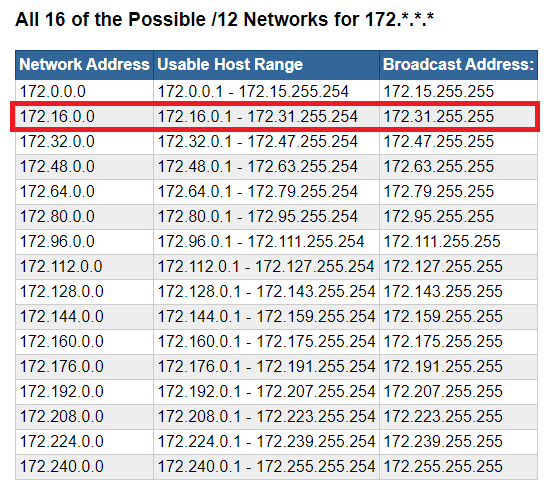172.16.0.0/12 highlighted in red

This means that the remainder of networks in the `172.0.0.0/12` address space are internet-routable address blocks.

If you haven't noticed yet, the network address in the picture above increases in multiples of 16 -- 0, 16, 32, 48, 64, and so on. This is because our network mask is `11110000`

Given a subnet mask, you can calculate the total number of possible networks the following ways:

1. Find the partial network mask
• A fully masked network ID is 8 bits -- `11111111`
• `/8``/16``/24``/32` represent CIDR masks where network ID octets are fully masked
• `11111111.00000000.00000000.00000000``/8`
• `11111111.11111111.00000000.00000000``/16`
• `11111111.11111111.11111111.00000000``/24`
• `/12` is between `/8` and `/16`
• `11111111.11110000.00000000.0000000`
full          partial         host         host
2. Then...
• Use the decimal value of the network mask
• Or, use the number of host bits as an exponent

Decimal Value

• `11110000` (base 2) = `240` (base 10)
• Total of 8 bits in a binary octet, with `256` possible values in decimal (as discussed earlier)
• `256``240``16`

Host Bit Exponent

• There are 4 bits in the host portion of the subnet mask
• Binary is base 2, so 2= 16
• Again, is the exponent here, as that's the number of host bits
• The total possible values in binary, you'll recall, are `1` or `0`, or base 2.
• 24 = 16, hence the network ID increases in multiples of 16

`172.0.x.x` to `172.15.x.x` -- 0, 1, 2, 3, 4, 5, 6, 7, 8, 9, 10, 11, 12, 13, 14, 15 -- 16 possible values (including zero)
`172.16.x.x` to `172.31.x.x` -- 16, 17, 18, 19, 20, 21, 22, 23, 24, 25, 26, 27, 28, 29, 30, 31 -- 16 possible values
Etc...

We'll get more practice with this a bit more down the page

Conversely, any octet where the network mask is `11111111` is fully masked, and the value can only be one value from `0` to `255`

192.168.0.0/16

Technically speaking, if there's a `192.168.0.0/16` , there is a `192.0.0.0/8` , as demonstrated in the screenshot below.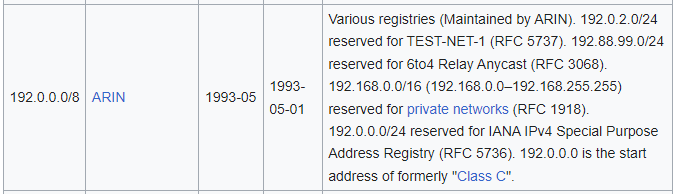https://en.wikipedia.org/wiki/List_of_assigned_/8_IPv4_address_blocks

Effectively, RFC 1918 has shrunk the `/8` to `/16` and said, that any address falling in `192.168.0.0/16` should be considered non-internet-routable (private).

``````192      . 168      . 0-255    . 0-254
255      . 255      . 0        . 0        = /16
11111111 . 11111111 . 00000000 . 00000000 = 16 bits on (1 x 16)
^          ^          ^          ^
|          |          |          |
Network     Network     Host       Host
|          |
|__________|
|
|
|____ Both of these octets are masked by 11111111
Therefore, both of these octets are static
at 192 and 168 respectively``````

All of this is important, because every device with a network interface card (NIC) is going to have a routing table:

• Hosts need to know what their Local Area Network (LAN) and what constitutes a foreign network
• Can the host put the packet on the wire and let the switch take care of it?
• Or, does it need to send it to the default gateway to be routed somewhere elese?
• Routers need to be able to know how to move packets

## Subnetting

DON'T OVERTHINK IT!

Folks spend a lot of time upskilling in the HOW of subnetting, but I don't think training materials really spend enough time on the WHY of subnetting.

### Why Do We Subnet?

A) To shrink the size of a network
B) To make multiple networks from a single network block

#### Scenario A: Shrink the `192.168.0.0/16` network

• RFC 1918 has allocated the `192.168.0.0/16` address block for use in private computer networking
• A `/16` network will yield 65,534 possible addresses.
• There's no way as residential users that we're going to use all of these addresses internally
``````192      . 168      . 0        . 0

255      . 255      . 0        . 0
11111111 . 11111111 . 00000000 . 00000000 = /16
^          ^           ^          ^
|          |           |          |
Network    Network      Host        Host
``````

Before

• We can "borrow bits" from the host segment and switch them to `1` to make them a network segment
• In this example, since we flipped all of the bits to `11111111`, we can use any value between `0` and `255`
• We've add eight more bits to the network mask, making it a `/24` network
• `192.168.1.0/24` and `192.168.0.0/24` are very common subnets shipped on consumer-grade routers.
``````192      . 168      . 0-255    . 1-254

255      . 255      . 255      . 0
11111111 . 11111111 . 11111111 . 00000000 = /24
^          ^           ^          ^
|          |           |          |
Network    Network     Network     Host
|          |
|          |______ This is the host octet now
|                  You can address any host from 1 through 254
|
|
|______ Choose a number between 0 through 255
However, this now becomes part of the network ID
So, this number is static, whichever you choose
``````

After

#### Scenario B: Increase the number of networks

Context

• You are working at an office branch and you are the network administrator
• The network engineer at HQ gave you a `10.10.0.0/16` address space to work with
• On its own, this address space yields:
• 1 possible network
• 65,534 possible hosts
• You're required to work with this space and have no control over additional networks
• You don't want to create routing conflicts with other branches in the organization
• You control `10.10.0.0/16` and any subvariant of this address space

The Problem

• You need to break this up into smaller chunks for multiple departments:
• Sales
• HR
• Engineering
• Once you've broken it up, you'll give this information to the network engineer, so that they can set up routes and firewall rules accordingly
``````10       . 10       . 0        . 0

255      . 255      . 0        . 0
11111111 . 11111111 . 00000000 . 00000000 = /16
^          ^          ^          ^
|          |          |          |
Network    Network      Host       Host
``````

The Solution

• We know we need 3 networks
• We need to calculate the number of host bits to borrow for the network mask.
• The formula to check this is 2nwhere `n` is the number of bits borrowed
• 21 = 2, meaning 2 subnets are possible if we borrow 1 bit; not enough
• 22 = 4, meaning 4 subnets are possible if we borrow 2 bits; more than we need, but the best possible choice
``````10       . 10       . 0         . 0

255      . 255      . 192       . 0
11111111 . 11111111 . 110000000 . 00000000 = /18
^          ^          ^          ^
|          |          |          |
Network    Network      Host       Host
``````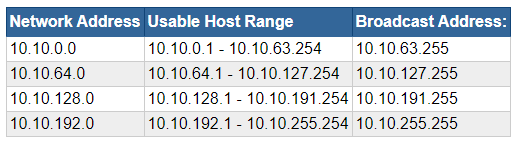### More Subnetting Practice

#### Shrink a Network Block

Scenario 1: Only 254 hosts per network

Problem

• You're setting up a lab network and you've decided to use the `10.0.0.0/8` RFC 1918 spaces
• In it's current state, you've got the following network mask
``````10       . 0        . 0        . 0

11111111 . 00000000 . 00000000 . 00000000
Network      Host       Host       Host

8  network bits
24 host bits
``````
• 224 = 16,777,216 = total addresses
• (224) - 2 = 16,777,214 = total usable addresses (minus network and broadcast)

Calculate the Number of Usable Host Bits

• (28) - 2 = 254 = total usable addresses = only 8 host bits
• We know there's a total of 32 bits in the network mask
• 32 total bits - 8 host bits = 24 = number of network bits
• Yielding network mask of `11111111.11111111.11111111.00000000`

Number of Usable Networks

``````10       . 0-255    . 0-255    . 1-254

11111111 . 11111111 . 11111111 . 00000000
Network  . Network  . Network  . Hosts
|          |          |
|          |          |____ Fully masked by 11111111
|          |                Which ever decimal value we set it to is static
|          |                Can choose any decimal value between 0-255
|          |                This will be the network ID
|          |
|          |____ Fully masked by 11111111
|                Which ever decimal value we set it to is static
|                Can choose any decimal value between 0-255
|                This will be the network ID
|
This value cannot be anything other than 10
The 10.0.0.0/8 network is the only "10" IP address block
Reserved by RFC 1918 for private networking``````
• `10` cannot be changed
• The second octet is variable and open to our choosing -- 8 bits
• The third octet is variable and open to our choosing -- 8 bits
• 8 bits + 8 bits = 16 bits
• 216 = 65,536 possible networks, with each network capable of having 254 hosts

Example

`10.1.2.0/24`

``````10       . 1        . 2        . 1-254

255      . 255      . 255      . 0
11111111 . 11111111 . 11111111 . 00000000 = /24
Network    Network    Network    Hosts

10.1.2 - are the network ID and are static

The last octet can be any value between 1-254 as a usable address``````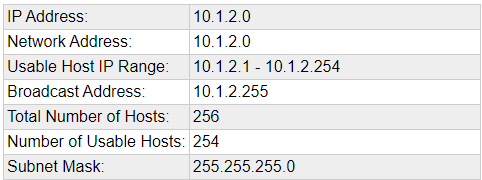Scenario 2: Only 30 hosts per network

Problem

• You're setting up a lab network and you've decided to use the `172.16.0.0/12` RFC 1918 space
• In it's current state, you've got the following network mask
``````172       . 16-31   . 0        . 0

11111111 . 11110000 . 00000000 . 00000000
Network    '-''---'    Host       Host       Host
Net Host

12  network bits
20 host bits
``````
• 220 = 1,048,576 = total addresses
• (220) - 2 = 1,048,574 = total usable addresses (minus network and broadcast)

Calculate the Number of Usable Host Bits

• (25) - 2 = 30 = total usable addresses = only 5 host bits
• We know there's a total of 32 bits in the network mask
• 32 total bits - 5 host bits = 27 = number of network bits
• Yielding network mask of `11111111.11111111.11111111.11100000`

Number of Usable Networks

``````172      . 16-31    . 0-255    . 1-254

11111111 . 11111111 . 11111111 . 11100000
Network  . Network  . Network  . '-''---'
|         |          |        |   |
|         |          |       Net  Hosts
|         |          |
|         |          |____ Fully masked by 11111111
|         |                Which ever decimal value we set it to is static
|         |                Can choose any decimal value between 0-255
|         |                This will be the network ID
|         |
|         |____ Per RFC 1918, this octet must be
|               Any deciaml value of 16 through 31
|
|____ Per RFC 1918, this octet may only be 172``````
• `172` cannot be changed
• The second octet may be between `16` and `31` per RFC 1918
• The third octet is variable and open to our choosing -- 8 bits
• We've got a total of 27 bits in the network mask -- `/27`
• The original network mask is 12 bits -- `/12`
• 27 - 12 = 15
• 215 = 32,768 possible networks, with each network capable of having 30 hosts

Example

`172.20.234.0/27`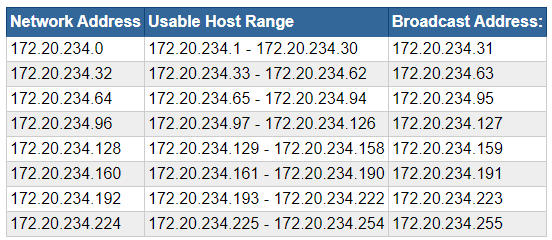#### Break up a Network Block

Scenario 1: Yield 3 Networks

Problem

• You've been given a `192.168.1.0/24` network to work with
• You need to divide this network up into three subnets

Convert the Subnet Mask to Binary

• `/24` means 24 bits are flipped on in the subnet mask
• `11111111.11111111.11111111.00000000` (1 x 24 = 16)
Network    Network   Network   Host

Calculate the Number of Host Bits to Borrow

• 2formula where `n` is the number of bits to borrow
• 21 = 2 networks, which does not yield enough networks for us, as we need 4
• 22 = 4 networks, which is more than we need, but the best we can do given the circumstances
``````Network Mask

255      . 255      . 255      . 192

11111111 . 11111111 . 11100000 . 11000000 = /26 (1 x 26)
Network .  Network .  Network . --'----'
||   |
Network  Host``````
• Our new CIDR notation is now `/26` (1 x 26)
• `192.168.1.0/26`

Calculate the Network Size

• 32 bits in a network mask
• 32 - 26 = 6 host bits
• 26 = 64 hosts per network

Calculate the Valid Decimal Values

``````192      . 168      . 1        . 0

255      . 255      . 255      . 192

11111111 . 11111111 . 11111111 . 11000000 = /26 (1 x 26)
Network .  Network .  Network . --'----'
|         |         |        ||   |
|         |         |    Network  Host
|         |         |             |
|         |         |             |____ 6 host bits = 2^6 = 64
|         |         |                   64 total addresses per network
|         |         |                   Increments of 64
|         |         |
|         |         |
|         |         |____ Fully masked by 11111111
|         |               This octet is static at
|         |                   1
|         |
|         |_____ Fully masked by 11111111
|                This octet is static at
|                    168
|
This octet is static at
192``````

Note that the network ID address increases by increments of 64

Scenario 2: Yield 8 Networks

Problem

• You've been given a `10.12.0.0/16` network to work with
• You need to divide this network up into eight subnets

Convert the Subnet Mask to Binary

• `/16` means 16 bits are flipped on in the subnet mask
• `11111111.11111111.00000000.00000000` (1 x 16 = 16)
Network    Network   Host         Host

Calculate the Number of Host Bits to Borrow

• 2formula where `n` is the number of bits to borrow
• 2= 8, therefore we need to borrow 3 bits from the host portion of the subnet mask
``````Network Mask

255      . 255      . 224      . 0

11111111 . 11111111 . 11100000 . 00000000 = /19 (1 x 19)
Network .  Network . '-''---' . Host
|   |
Network  Host``````
• Our new CIDR notation is now `/19` (1 x 19)
• `10.12.0.0/19`

Calculate the Network Size

• 32 bits in a network mask
• 32 - 19 = 13 host bits
• 213 = 8,192 hosts per network

Calculate the Valid Decimal Values

``````10       . 12       . 0        . 0

255      . 255      . 224      . 0

11111111 . 11111111 . 11100000 . 00000000 = /19 (1 x 19)
Network .  Network . '-''---' . Host
|   |       |
Network  Host    |____ 8 host bits
|             2^8 = 0-255
|
|
|_____ 5 host bits
2^5 = 0-31
Increments of 32``````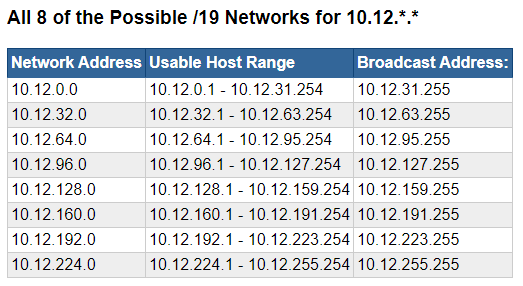Note that the network ID address increases by increments of 32